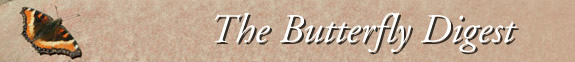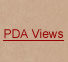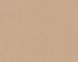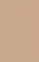Jump to : Today June 3, 2023 June 2, 2023 June 1, 2023 May 31, 2023 May 30, 2023 May 29, 2023 May 28, 2023 May 27, 2023 May 26, 2023 May 25, 2023 May 24, 2023 May 23, 2023 May 22, 2023 May 21, 2023 May 20, 2023 May 19, 2023 May 18, 2023 May 17, 2023 May 16, 2023 May 15, 2023 May 14, 2023 May 13, 2023 May 12, 2023 May 11, 2023 May 10, 2023 May 9, 2023 May 8, 2023 May 7, 2023 May 6, 2023 May 5, 2023     |    View All Lists    |    FAQ
 List: MassLep Date: Mon, 8 May 2023 19:36:36 -0400 From: Barbara Volkle Subject: [MassLep] local sightings - 5/7 and 5/8 Here are some sightings from our local patch.Steve Moore and Barbara VolkleNorthborough, MA*Sunday, May 7 - Oxbow NWR, Harvard =C2=A0=C2=A0=C2=A0=C2=A0=C2=A0 Eastern Pine Elfin 1 =C2=A0=C2=A0=C2=A0=C2=A0=C2=A0 Cabbage White 1 =C2=A0=C2=A0=C2=A0=C2=A0=C2=A0 American Lady 1 =C2=A0=C2=A0=C2=A0=C2=A0=C2=A0 Mourning Cloak 1 =C2=A0=C2=A0=C2=A0=C2=A0=C2=A0 Eastern Tiger Swallowtail 1 =C2=A0=C2=A0=C2=A0=C2=A0 Cabbage White 4 - at the Dairy Queen in ClintonSunday, May 7 - Northborough =C2=A0=C2=A0=C2=A0=C2=A0 Red Admiral 1 =C2=A0=C2=A0=C2=A0=C2=A0 Eastern Tiger Swallowtail 1 =C2=A0=C2=A0 =C2=A0 Mourning Cloak 1Monday, May 8 - Lake Chauncy, Westborough =C2=A0=C2=A0=C2=A0=C2=A0 Eastern Comma 1 =C2=A0=C2=A0=C2=A0=C2=A0 Eastern Tiger Swallowtail 3 =C2=A0=C2=A0 =C2=A0 Eastern Comma 1 =C2=A0=C2=A0=C2=A0=C2=A0 Clouded Sulphur 2 =C2=A0=C2=A0=C2=A0=C2=A0 Spring Azure 1 =C2=A0=C2=A0=C2=A0=C2=A0 Cabbage White 7Sunday, May 8 - Northborough =C2=A0=C2=A0=C2=A0=C2=A0 Eastern Comma 1--=20You received this message because you are subscribed to the Google Groups "=MassLep" group.To unsubscribe from this group and stop receiving emails from it, send an e=mail to masslep+To view this discussion on the web visit https://groups.google.com/d/msgid/=masslep/<70b3c8a7-5ba6-6661-6c06-7321ce2fe313...>